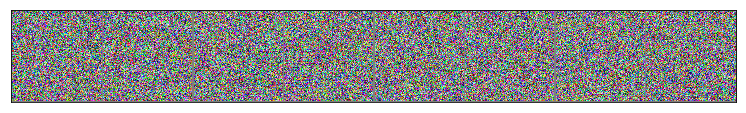# Extending datasets in pyTorch

PyTorch is a great library for machine learning. You can in a few lines of codes retrieve a dataset, define your model, add a cost function and then train your model. It's quite magic to copy and paste code from the internet and get the LeNet network working in a few seconds to achieve more than 98% accuracy.

However, it can be tedious sometimes to extend existing objects and here, I will manipulate some ways to define the right dataset for your application. In particular I will modify the call to a standard dataset (MNIST) to place the characters at random places in a large image.

Let's first initialize the notebook:

In :
import numpy as np
np.set_printoptions(precision=6, suppress=True)
import os
%matplotlib inline
import matplotlib.pyplot as plt


We will use the Dataset object https://pytorch.org/docs/stable/data.html and the MNIST example from the torchvision datasets.

In :
from torchvision.datasets import MNIST
import torch
from torchvision import datasets, transforms

mnist = datasets.MNIST('/tmp/data',
transform=transforms.Compose([
transforms.ToTensor(),
transforms.Normalize((0.1307,), (0.3081,))]))
batch_size=8,
shuffle=True)

from torchvision.utils import make_grid
import matplotlib.pyplot as plt
fig, ax = plt.subplots(figsize=((13, 5)))
import numpy as np
ax.imshow(np.transpose(npimg, (1, 2, 0)))
plt.setp(ax, xticks=[], yticks=[])

return fig, ax
plt.show()It's easy to define some geometrical transforms such as cropping:

In :
mnist = datasets.MNIST('/tmp/data',
transform=transforms.Compose([
transforms.CenterCrop(18),
transforms.ToTensor(),
transforms.Normalize((0.1307,), (0.3081,))]))
batch_size=8,
shuffle=True)

plt.show()... or a zoom out (by cropping by a size bigger than the input size, which seems unintuitive at first):

In :
from torchvision.datasets import MNIST
import torch
from torchvision import datasets, transforms

mnist = datasets.MNIST('/tmp/data',
transform=transforms.Compose([
transforms.CenterCrop(128),
transforms.ToTensor(),
transforms.Normalize((0.1307,), (0.3081,))]))
batch_size=8,
shuffle=True)

plt.show()In :
torch.Tensor

Out:
torch.Tensor

The code for defining this particular dataset is available :

https://github.com/pytorch/vision/blob/master/torchvision/datasets/mnist.py

and can be a great inspiration to see how the object is defined... in this particular case, we wish to translate the MNIST image in the full scale image.

In :
datasets.MNIST?

Init signature:
datasets.MNIST(
root,
train=True,
transform=None,
target_transform=None,
)
Docstring:
MNIST <http://yann.lecun.com/exdb/mnist/>_ Dataset.

Args:
root (string): Root directory of dataset where processed/training.pt
and  processed/test.pt exist.
train (bool, optional): If True, creates dataset from training.pt,
otherwise from test.pt.
transform (callable, optional): A function/transform that  takes in an PIL image
and returns a transformed version. E.g, transforms.RandomCrop
target_transform (callable, optional): A function/transform that takes in the
target and transforms it.
File:           /usr/local/lib/python3.7/site-packages/torchvision/datasets/mnist.py
Type:           type
Subclasses:     FashionMNIST, KMNIST, EMNIST


Moreover, thes following code is a great inspiration to generate arbitrary data:

https://github.com/pytorch/vision/blob/master/torchvision/datasets/fakedata.py

In :
class FakeData(torch.utils.data.Dataset):
"""A fake dataset that returns randomly generated images and returns them as PIL images
Args:
size (int, optional): Size of the dataset. Default: 1000 images
image_size(tuple, optional): Size if the returned images. Default: (3, 224, 224)
num_classes(int, optional): Number of classes in the datset. Default: 10
transform (callable, optional): A function/transform that  takes in an PIL image
and returns a transformed version. E.g, transforms.RandomCrop
target_transform (callable, optional): A function/transform that takes in the
target and transforms it.
random_offset (int): Offsets the index-based random seed used to
generate each image. Default: 0
"""

def __init__(self, size=1000, image_size=(3, 224, 224), num_classes=10,
transform=None, target_transform=None, random_offset=0):
self.size = size
self.num_classes = num_classes
self.image_size = image_size
self.transform = transform
self.target_transform = target_transform
self.random_offset = random_offset

def __getitem__(self, index):
"""
Args:
index (int): Index
Returns:
tuple: (image, target) where target is class_index of the target class.
"""
# create random image that is consistent with the index id
rng_state = torch.get_rng_state()
torch.manual_seed(index + self.random_offset)
img = torch.randn(*self.image_size)
target = torch.Tensor(1).random_(0, self.num_classes)
torch.set_rng_state(rng_state)

# convert to PIL Image
img = transforms.ToPILImage()(img)
if self.transform is not None:
img = self.transform(img)
if self.target_transform is not None:
target = self.target_transform(target)

return img, target

def __len__(self):
return self.size

def __repr__(self):
fmt_str = 'Dataset ' + self.__class__.__name__ + '\n'
fmt_str += '    Number of datapoints: {}\n'.format(self.__len__())
tmp = '    Transforms (if any): '
fmt_str += '{0}{1}\n'.format(tmp, self.transform.__repr__().replace('\n', '\n' + ' ' * len(tmp)))
tmp = '    Target Transforms (if any): '
fmt_str += '{0}{1}'.format(tmp, self.target_transform.__repr__().replace('\n', '\n' + ' ' * len(tmp)))
return fmt_str

mnist = FakeData(transform=transforms.Compose([
transforms.CenterCrop(128),
transforms.ToTensor(),
transforms.Normalize((0.1307,), (0.3081,))]))

In :
mnist

Out:
Dataset FakeData
Number of datapoints: 1000
Transforms (if any): Compose(
CenterCrop(size=(128, 128))
ToTensor()
Normalize(mean=(0.1307,), std=(0.3081,))
)
Target Transforms (if any): None
In :

batch_size=8,
shuffle=True)

plt.show()### a simpler way¶

One can also create a function and add it to the list of transforms:

In :
class z_score(object):
'''
Image per Image z-score normalization

Image = (Image-mean(Image))/std(Image)
'''
def __call__(self, img):
img = img - img.mean()
img = img / img.std()

return img

class rock_and_roll(object):
'''
Image per Image z-score normalization

Image = (Image-mean(Image))/std(Image)
'''
def __call__(self, img):

img = img.numpy()
x_roll , y_roll = int(np.random.rand()*img.shape), int(np.random.rand()*img.shape)
img = np.roll(img, x_roll, axis=1)
img = np.roll(img, y_roll, axis=2)
img = torch.Tensor(img)

return img


In :
from torchvision.datasets import MNIST
import torch
from torchvision import datasets, transforms

mnist = datasets.MNIST('/tmp/data',
transform=transforms.Compose([
transforms.CenterCrop(128),
transforms.ToTensor(),
z_score(),
rock_and_roll(),
transforms.Normalize((0.1307,), (0.3081,))]))
batch_size=8,
shuffle=True)

plt.show()### some book keeping for the notebook¶

In :
%load_ext watermark
%watermark

2019-03-14T14:50:46+01:00

CPython 3.7.2
IPython 7.3.0

compiler   : Clang 10.0.0 (clang-1000.11.45.5)
system     : Darwin
release    : 18.2.0
machine    : x86_64
processor  : i386
CPU cores  : 36
interpreter: 64bit

In :
%load_ext version_information
%version_information numpy, matplotlib, torch

Out:
Software Version
Python 3.7.2 64bit [Clang 10.0.0 (clang-1000.11.45.5)]
IPython 7.3.0
OS Darwin 18.2.0 x86_64 i386 64bit
numpy 1.16.2
matplotlib 3.0.2
torch 1.0.1.post2
Thu Mar 14 14:50:46 2019 CET